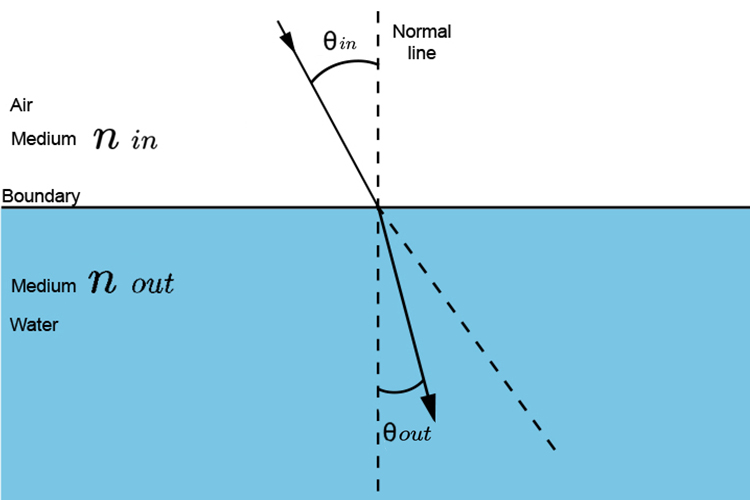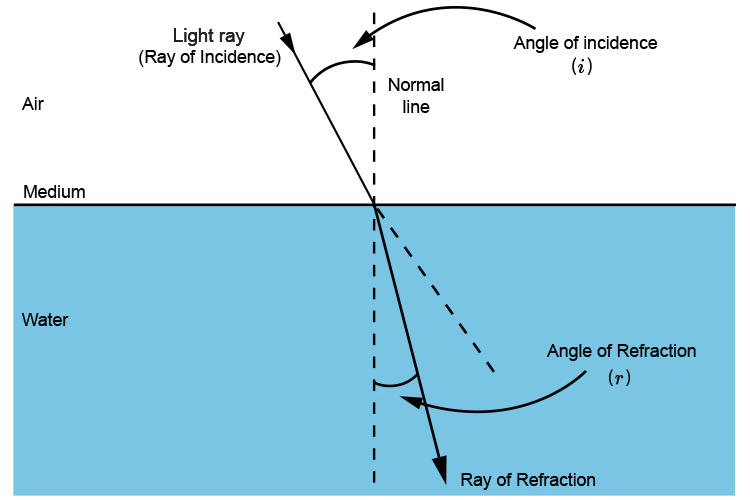# Snell's law in use

Snell's law can be simplified in use because in most text books the medium going in is air.NOTE: how theta relates to the angle going in against the normal line is different to the angle coming out against the normal line.

We have learnt that Snell's law is

(sin\ \i\n\ \theta)/(sin\ \out\ \theta)=(n\ \(out))/(n\ \(i\n))

But the refractive index n of air for all practical purposes is one (1).

(In fact a vacuum is 1 and air is 1.0002926)

So most text books show Snell's law as being

(sin\ \i\n\ \theta)/(sin\ \out\ \theta)=n\ \(out)Angle of the incident = Angle of incidence (i)

Angle refracted = Angle of refraction (r)

Snell's law becomes:

(sin\theta\i)/(sin\theta\r)=(n\ \m\e\d\i\u\m\ \w\a\t\e\r)/(n\ \m\e\d\i\u\m\ \a\i\r)

Which becomes

(sin\theta\i)/(sin\theta\r)=n/1

Which becomes

(sin\theta\i)/(sin\theta\r)=n (refractive index of medium)

## Summary

Most text books show Snell's law as

n=(sin\ \i)/(sin\ \r)